# Difference between revisions of "Free magma"

Letbe a set. Define sets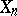,, inductively as follows: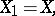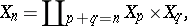wheredenotes the disjoint union (see Union of sets). LetThere is an obvious binary operation on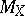: if,, then the pair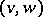goes to the elementof. This is the free magma on. It has the obvious freeness property: ifis any magma and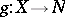is a function, then there is a unique morphism of magmas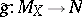extending.

Certain special subsets of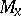, called Hall sets (cf. Hall set), are important in combinatorics and the theory of Lie algebras.

The free magma overcan be identified with the set of binary complete, planar, rooted trees with leaves labelled by. See Binary tree.

How to Cite This Entry:
Free magma. Encyclopedia of Mathematics. URL: http://encyclopediaofmath.org/index.php?title=Free_magma&oldid=12862
This article was adapted from an original article by M. Hazewinkel (originator), which appeared in Encyclopedia of Mathematics - ISBN 1402006098. See original article